# Training and Testing a Neural Network for LLR Estimation

This example shows how to generate signals and channel impairments to train a neural network, called LLRNet, to estimate exact log likelihood ratios (LLR).

Most modern communication systems, such as 5G New Radio (NR) and Digital Video Broadcasting for Satellite, Second Generation (DVB-S.2) use forward error correction algorithms that benefit from soft demodulated bit values. These systems calculate soft bit values using the LLR approach. LLR is defined as the log of the ratio of probability of a bit to be 0 to the probability of a bit to be 1 or

${l}_{i}\triangleq \mathrm{log}\left(\frac{{P}_{r}\left({c}_{i}=0|\underset{}{\overset{ˆ}{s}}\right)}{{P}_{r}\left({c}_{i}=1|\underset{}{\overset{ˆ}{s}}\right)}\right)$, $i=1,...,k$

where $\underset{}{\overset{ˆ}{s}}$ is an k-bit received symbol, and ${c}_{i}$ is the ${i}^{th}$bit of the symbol. Assuming an additive white Gaussian noise (AWGN) channel, the exact computation of the LLR expression is

`${l}_{i}\triangleq \mathrm{log}\left(\frac{\sum _{s\in {c}_{i}^{0}}\mathrm{exp}\left(-\frac{‖\underset{}{\overset{ˆ}{s}}-s{‖}_{2}^{2}}{{\sigma }^{2}}\right)}{\sum _{s\in {c}_{i}^{1}}\mathrm{exp}\left(-\frac{‖\underset{}{\overset{ˆ}{s}}-s{‖}_{2}^{2}}{{\sigma }^{2}}\right)}\right)$`

where ${\sigma }^{2}$ is the noise variance. Exponential and logarithmic calculations are very costly especially in embedded systems. Therefore, most practical systems use the max-log approximation. For a given array $\mathit{x}$, the max-log approximation is

$\mathrm{log}\left(\sum _{j}\mathrm{exp}\left(-{x}_{j}^{2}\right)\right)\approx \underset{j}{\mathrm{max}}\left(-{x}_{j}^{2}\right)$.

Substituting this in the exact LLR expression results in the max-log LLR approximation 

${l}_{i}\approx \frac{1}{{\sigma }^{2}}\left(\underset{s\in {C}_{i}^{1}}{\mathrm{min}}‖\underset{}{\overset{ˆ}{s}}-s{‖}_{2}^{2}-\underset{s\in {C}_{i}^{0}}{\mathrm{min}}‖\underset{}{\overset{ˆ}{s}}-s{‖}_{2}^{2}\right)$.

LLRNet uses a neural network to estimate the exact LLR values given the baseband complex received symbol for a given SNR value. A shallow network with a small number of hidden layers has the potential to estimate the exact LLR values at a complexity similar to the approximate LLR algorithm .

### Compare Exact LLR, Max-Log Approximate LLR and LLRNet for M-ary QAM

5G NR uses M-ary QAM modulation. This section explores the accuracy of LLRNet in estimating the LLR values for 16-, 64-, and 256-QAM modulation. Assume an M-ary QAM system that operates under AWGN channel conditions. This assumption is valid even when the channel is frequency selective but symbols are equalized. The following shows calculated LLR values for the following three algorithms:

• Exact LLR

• Max-log approximate LLR

• LLRNet

#### 16-QAM LLR Estimation Performance

Calculate exact and approximate LLR values for symbol values that cover the 99.7% ($±3\sigma$) of the possible received symbols. Assuming AWGN, 99.7% ($±3\sigma$) of the received signals will be in the range $\left[\underset{s\in C}{\mathrm{max}}\left(Re\left(s\right)+3\sigma \right)\underset{s\in C}{\mathrm{min}}\left(Re\left(s\right)-3\sigma \right)\right]+i\left[\underset{s\in C}{\mathrm{max}}\left(Im\left(s\right)+3\sigma \right)\underset{s\in C}{\mathrm{min}}\left(Im\left(s\right)-3\sigma \right)\right]$. Generate uniformly distributed I/Q symbols over this space and use `qamdemod` (Communications Toolbox) function to calculate exact LLR and approximate LLR values.

```M = 16; % Modulation order k = log2(M); % Bits per symbols SNRValues = -5:5:5; % in dB numSymbols = 1e4; numSNRValues = length(SNRValues); symOrder = llrnetQAMSymbolMapping(M); const = qammod(0:15,M,symOrder,'UnitAveragePower',1); maxConstReal = max(real(const)); maxConstImag = max(imag(const)); numBits = numSymbols*k; exactLLR = zeros(numBits,numSNRValues); approxLLR = zeros(numBits,numSNRValues); rxSym = zeros(numSymbols,numSNRValues); for snrIdx = 1:numSNRValues SNR = SNRValues(snrIdx); noiseVariance = 10^(-SNR/10); sigma = sqrt(noiseVariance); maxReal = maxConstReal + 3*sigma; minReal = -maxReal; maxImag = maxConstImag + 3*sigma; minImag = -maxImag; r = (rand(numSymbols,1)*(maxReal-minReal)+minReal) + ... 1i*(rand(numSymbols,1)*(maxImag-minImag)+minImag); rxSym(:,snrIdx) = r; exactLLR(:,snrIdx) = qamdemod(r,M,symOrder,... 'UnitAveragePower',1,'OutputType','llr','NoiseVariance',noiseVariance); approxLLR(:,snrIdx) = qamdemod(r,M,symOrder,... 'UnitAveragePower',1,'OutputType','approxllr','NoiseVariance',noiseVariance); end```

#### Set Up and Train Neural Network

Set up a shallow neural network with one input layer, one hidden layer, and one output layer. Input a received symbol to the network and train it to estimate the exact LLR values. Since the network expects real inputs, create a two column vector, where the first column is the real values of the received symbol and the second column is the imaginary values of the received symbol. Also, the output must be a $\mathit{k}×\mathit{N}$ vector, where $\mathit{k}$ is the number of bits per symbol and $\mathit{N}$ is the number of symbols.

```nnInput = zeros(numSymbols,2,numSNRValues); nnOutput = zeros(numSymbols,k,numSNRValues); for snrIdx = 1:numSNRValues rxTemp = rxSym(:,snrIdx); rxTemp = [real(rxTemp) imag(rxTemp)]; nnInput(:,:,snrIdx) = rxTemp; llrTemp = exactLLR(:,snrIdx); nnOutput(:,:,snrIdx) = reshape(llrTemp, k, numSymbols)'; end```

For 16-QAM symbols, the hidden layer has 8 neurons and the output layer has 4 neurons, which corresponds to the number of bits per symbol. The llrnetNeuralNetwork function returns a preconfigured neural network. Train the neural network for three different SNR values. Use the exact LLR values calculated using the `qamdemod` function as the expected output values.

```hiddenLayerSize = 8; trainedNetworks = cell(1,numSNRValues); for snrIdx=1:numSNRValues fprintf('Training neural network for SNR = %1.1fdB\n', ... SNRValues(snrIdx)) x = nnInput(:,:,snrIdx)'; y = nnOutput(:,:,snrIdx)'; MSExactLLR = mean(y(:).^2); fprintf('\tMean Square LLR = %1.2f\n', MSExactLLR) % Train the Network. Use parallel pool, if available. Train three times % and pick the best one. mse = inf; for p=1:3 netTemp = llrnetNeuralNetwork(hiddenLayerSize); if parallelComputingLicenseExists() [netTemp,tr] = train(netTemp,x,y,'useParallel','yes'); else [netTemp,tr] = train(netTemp,x,y); end % Test the Network predictedLLRSNR = netTemp(x); mseTemp = perform(netTemp,y,predictedLLRSNR); fprintf('\t\tTrial %d: MSE = %1.2e\n', p, mseTemp) if mse > mseTemp mse = mseTemp; net = netTemp; end end % Store the trained network trainedNetworks{snrIdx} = net; fprintf('\tBest MSE = %1.2e\n', mse) end```
```Training neural network for SNR = -5.0dB ```
``` Mean Square LLR = 4.43 ```
``` Trial 1: MSE = 7.44e-05 Trial 2: MSE = 6.90e-05 Trial 3: MSE = 6.81e-05 ```
``` Best MSE = 6.81e-05 ```
```Training neural network for SNR = 0.0dB ```
``` Mean Square LLR = 15.74 ```
``` Trial 1: MSE = 1.86e-03 Trial 2: MSE = 4.04e-04 Trial 3: MSE = 9.05e-05 ```
``` Best MSE = 9.05e-05 ```
```Training neural network for SNR = 5.0dB ```
``` Mean Square LLR = 60.01 ```
``` Trial 1: MSE = 8.59e-03 Trial 2: MSE = 2.19e-02 Trial 3: MSE = 2.14e-02 ```
``` Best MSE = 8.59e-03 ```

Performance metric for this network is mean square error (MSE). The final MSE values show that the neural network converges to an MSE value that is at least 40 dB less than the mean square exact LLR values. Note that, as SNR increases so do the LLR values, which results in relatively higher MSE values.

#### Results for 16-QAM

Compare the LLR estimates of LLRNet to that of exact LLR and approximate LLR. Simulate 1e4 16-QAM symbols and calculate LLR values using all three methods. Do not use the symbols that we generated in the previous section so as not to give LLRNet an unfair advantage, since those symbols were used to train the LLRNet.

```numBits = numSymbols*k; d = randi([0 1], numBits, 1); txSym = qammod(d,M,symOrder,'InputType','bit','UnitAveragePower',1); exactLLR = zeros(numBits,numSNRValues); approxLLR = zeros(numBits,numSNRValues); predictedLLR = zeros(numBits,numSNRValues); rxSym = zeros(length(txSym),numSNRValues); for snrIdx = 1:numSNRValues SNR = SNRValues(snrIdx); sigmas = 10^(-SNR/10); r = awgn(txSym,SNR); rxSym(:,snrIdx) = r; exactLLR(:,snrIdx) = qamdemod(r,M,symOrder,... 'UnitAveragePower',1,'OutputType','llr','NoiseVariance',sigmas); approxLLR(:,snrIdx) = qamdemod(r,M,symOrder,... 'UnitAveragePower',1,'OutputType','approxllr','NoiseVariance',sigmas); net = trainedNetworks{snrIdx}; x = [real(r) imag(r)]'; tempLLR = net(x); predictedLLR(:,snrIdx) = reshape(tempLLR, numBits, 1); end qam16Results.exactLLR = exactLLR; qam16Results.approxLLR = approxLLR; qam16Results.predictedLLR = predictedLLR; qam16Results.RxSymbols = rxSym; qam16Results.M = M; qam16Results.SNRValues = SNRValues; qam16Results.HiddenLayerSize = hiddenLayerSize; qam16Results.NumSymbols = numSymbols;```

The following figure shows exact LLR, max-log approximate LLR, and LLRNet estimate of LLR values versus the real part of the received symbol for odd bits. LLRNet matches the exact LLR values even for low SNR values.

`llrnetPlotLLR(qam16Results,'16-QAM LLR Comparison')`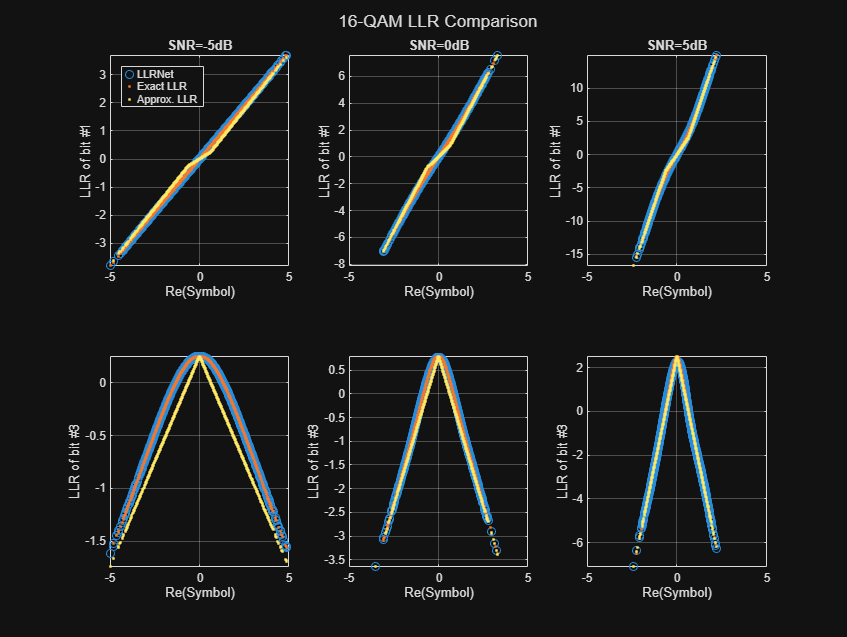#### 64-QAM and 256-QAM LLR Estimation Performance

Check if the LLRNet can estimate the LLR values for higher order QAM. Repeat the same process you followed for 16-QAM for 64-QAM and 256-QAM using the llrnetQAMLLR helper function. The following figures show exact LLR, max-log approximate LLR, and LLRNet estimate of LLR values versus the real part of the received symbol for odd bits.

```trainNow =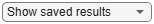false; if trainNow % Parameters for 64-QAM simParams(1).M = 64; %#ok<UNRCH> simParams(1).SNRValues = 0:5:10; simParams(1).HiddenLayerSize = 16; simParams(1).NumSymbols = 1e4; simParams(1).UseReLU = false; % Parameters for 256-QAM simParams(2).M = 256; simParams(2).SNRValues = 0:10:20; simParams(2).HiddenLayerSize = 32; simParams(2).NumSymbols = 1e4; simParams(2).UseReLU = false; simResults = llrnetQAMLLR(simParams); llrnetPlotLLR(simResults(1),sprintf('%d-QAM LLR Comparison',simResults(1).M)) llrnetPlotLLR(simResults(2),sprintf('%d-QAM LLR Comparison',simResults(2).M)) else load('llrnetQAMPerformanceComparison.mat', 'simResults') for p=1:length(simResults) llrnetPlotLLR(simResults(p),sprintf('%d-QAM LLR Comparison',simResults(p).M)) end end```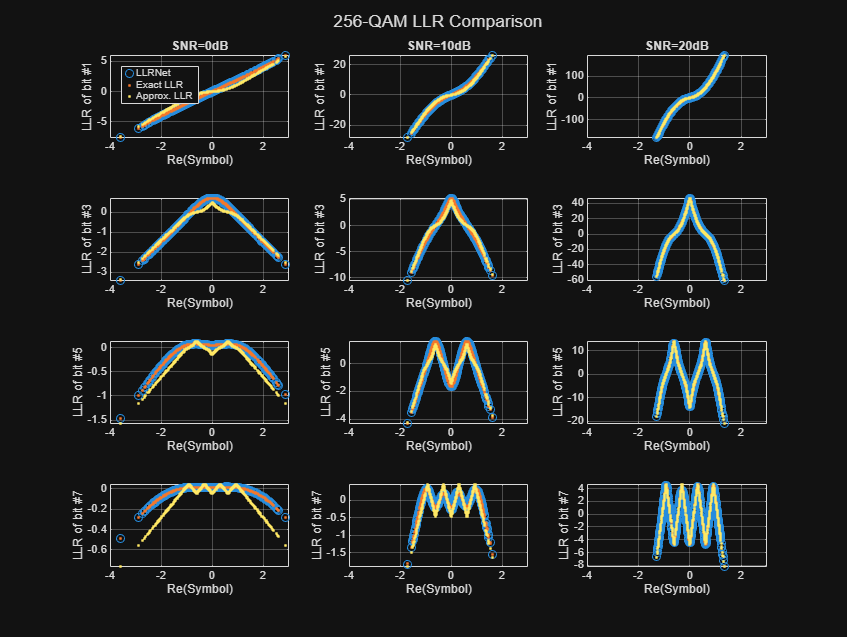### DVB-S.2 Packet Error Rate

DVB-S.2 system uses a soft demodulator to generate inputs for the LDPC decoder. Simulate the packet error rate (PER) of a DVB-S.2 system with 16-APSK modulation and 2/3 LDPC code using exact LLR, approximate LLR, and LLRNet using llrNetDVBS2PER function. This function uses the `comm.PSKDemodulator` (Communications Toolbox) System object™ and the `dvbsapskdemod` (Communications Toolbox) function to calculate exact and approximate LLR values and the `comm.AWGNChannel` (Communications Toolbox) System object to simulate the channel.

Set `simulateNow` to true (or select "Simulate" in the dropdown) to run the PER simulations for the values of `subsystemType`, `EsNoValues`, and `numSymbols` using the llrnetDVBS2PER function. If Parallel Computing Toolbox™ is installed, this function uses the `parfor` command to run the simulations in parallel. On an Intel® Xeon® W-2133 CPU @ 3.6GHz and running a Run Code on Parallel Pools (Parallel Computing Toolbox) of size 6, the simulation takes about 40 minutes. Set `simulateNow` to `false` (or select "Plot saved results" in the dropdown), to load the PER results for the values of `subsystemType`=`'16APSK 2/3'`, `EsNoValues`=`8.6:0.1:8.9`, and `numSymbols`=`10000`.

Set `trainNow` to `true` (or select "Train LLRNet" in the dropdown) to train LLR neural networks for each value of `EsNoValues`, for the given `subsystemType` and `numSymbols`. If Parallel Computing Toolbox is installed, the `train` function can be called with the optional name-value pair `'useParallel'` set to `'yes'` to run the simulations in parallel. On an Intel Xeon W-2133 CPU @ 3.6GHz and running a Run Code on Parallel Pools (Parallel Computing Toolbox) of size 6, the simulation takes about 21 minutes. Set `trainNow` to false (or select "Use saved networks" in the dropdown) to load LLR neural networks trained for `subsystemType`=`'16APSK 2/3'`, `EsNoValues`=`8.6:0.1:8.9`.

For more information on the DVB-S.2 PER simulation, see the DVB-S.2 Link, Including LDPC Coding in Simulink (Communications Toolbox) example. For more information on training the network, refer to the llrnetTrainDVBS2LLRNetwork function and .

```simulateNow =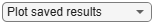false; if simulateNow subsystemType = '16APSK 2/3'; %#ok<UNRCH> EsNoValues = 8.6:0.1:8.9; % in dB numFrames = 10000; numErrors = 200; trainNow =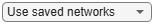false; if trainNow && (~strcmp(subsystemType,'16APSK 2/3') || ~isequal(EsNoValues,8.6:0.1:9)) % Train the networks for each EsNo value numTrainSymbols = 1e4; hiddenLayerSize = 64; llrNets = llrnetTrainDVBS2LLRNetwork(subsystemType, EsNoValues, numTrainSymbols, hiddenLayerSize); else load('llrnetDVBS2Networks','llrNets','subsystemType','EsNoValues'); end % Simulate PER with exact LLR, approximate LLR, and LLRNet [perLLR,perApproxLLR,perLLRNet] = llrnetDVBS2PER(subsystemType,EsNoValues,llrNets,numFrames,numErrors); llrnetPlotLLRvsEsNo(perLLR,perApproxLLR,perLLRNet,EsNoValues,subsystemType) else load('llrnetDVBS2PERResults.mat','perApproxLLR','perLLR','perLLRNet',... 'subsystemType','EsNoValues'); llrnetPlotLLRvsEsNo(perLLR,perApproxLLR,perLLRNet,EsNoValues,subsystemType) end```The results show that the LLRNet almost matches the performance of exact LLR without using any expensive operations such as logarithm and exponential.

### Further Exploration

Try different modulation and coding schemes for the DVB-S.2 system. The full list of modulation types and coding rates are given in the DVB-S.2 Link, Including LDPC Coding in Simulink (Communications Toolbox) example. You can also try different sizes for the hidden layer of the network to reduce the number of operations and measure the performance loss as compared to exact LLR.

The example uses these helper functions. Examine these files to learn about details of the implementation.

### References

 O. Shental and J. Hoydis, ""Machine LLRning": Learning to Softly Demodulate," 2019 IEEE Globecom Workshops (GC Wkshps), Waikoloa, HI, USA, 2019, pp. 1-7.